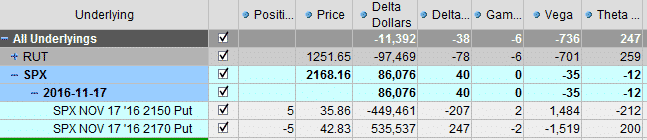## Contents

Day 1 of Bootcamp and today we’re looking at the Greeks and Greek Ratios.

Some of the info below might be obvious to some of you, but there is lots of good stuff if you keep reading to the end.

So, let’s get cracking.

DELTA –  Delta is the option’s sensitivity to changes in the underlying stock price. It measures the expected price change of the option.

Calls have a delta between 0 and 1
Puts have a delta between 0 and -1
Delta can be used as a “rough” probability

As an option goes further in the money, the likelihood that it will expire in the money increases. Therefore the Delta increases. Let’s look at an example:As expiration approaches, the stock will have less chance to move above or below the strike price. In-the-money options will rapidly approach 1 (calls) and -1 (puts) as expiration approaches.

Out-of-the-money options will rapidly move towards 0 as expiration approaches.GAMMA – The Gamma metric is the sensitivity of the delta to changes in price of the underlying asset. Gamma measures the change in the delta for a \$1 change in the underlying.

Gamma is Delta’s ugly cousin. Not many beginners pay attention to Gamma, and it can do serious damage to your portfolio.

If Delta is the “speed” at which option prices change, you can think of Gamma as the “acceleration”.

Options with the highest Gamma are the most responsive to changes in the price of the underlying stock.

HIGH GAMMA = BIG FLUCTUATIONS IN P&L

High Gamma can be a good thing or a bad thing. Positive Gamma is good if you get a big move (gains accelerate exponentially). Negative Gamma is bad if you get a big move (losses accelerate exponentially).

Some of you may have experienced this with Iron Condors that start moving against you.

Gamma is the highest close to expiry

You may have noticed this if you have traded weekly options. They call the last week of an options life “gamma week”.

Options have a very high price sensitivity when gamma is high.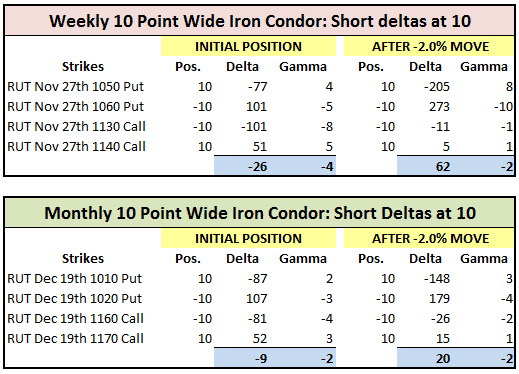Gamma Scalping is a technique used by market makers and other professional traders.

I’ll go in to this strategy in more detail later.

Gamma scalping requires traders to be well capitalized.

Traders also need to have a very good understanding of how option greeks work before undertaking this strategy.

VEGA – Vega is the option’s sensitivity to changes in implied volatility. A rise in implied volatility is a rise in option premiums, and so will increase the value of long calls and long puts. Vega increases with each expiration further out in time.

Typically, as implied volatility increases, the value of options will increase. That’s because an increase in implied volatility suggests an increased range of potential movement for the stock.

Here is a theoretical example to demonstrate the idea. Let’s look at a stock priced at 50. Consider a 6-month call option with a strike price of 50:

If the implied volatility is 90, the option price is \$12.50

If the implied volatility is 50, the option price is \$7.25

If the implied volatility is 30, the option price is \$4.50

RHO – Rho is the amount an option will change based on a one percentage point change in interest rates.

Most traders ignore Rho. If you’re trading short-term options, changes in interest rates will not have much of an impact.

The only options that tend to be materially impacted by interest rates are LEAPs due to the change in the “cost of carry”.

## Greek Ratios

There is no single best way to trade options. Neither is there a best way to manage the greeks.

The important thing to understand is the WHY we should pay attention to Greek Ratios and why we set certain guidelines in terms of Greek Ratios.

Another point to emphasize is not to get too hung up on specific Greek Ratios. There usually has to be some level of judgement used. Knowing some key ratios will help you know when to adjust and help avoid large losses.

## Delta Theta Ratio

The Delta Theta ratio is the most important Greek Ratio. It is literally the position Delta divided by Theta.

With options income trading, we are aiming to maximize Theta. We want time decay working in our favor.

If we want Theta to be the major driver in the trade, then we want Delta to be very low in proportion to Theta.

A high Delta to Theta ratio means price is the major factor in the trade, rather than Theta.

Greek Ratio guidelines will be different depending on the strategy and instrument traded.

For a typical Iron Condor that began with a Delta / Theta Ratio near 0%, you want adjust when the Delta / Theta Ratio gets to around 30%.

Again this is not a hard rule. Just know, that if you don’t adjust and the position continues to move against you, losses can accumulate very quickly.

Looking at this position, you can see that the condor is no longer Delta Neutral as the market has moved up.

The Delta Theta Ratio is 33%. Calculate as 38 / 115 = 33%.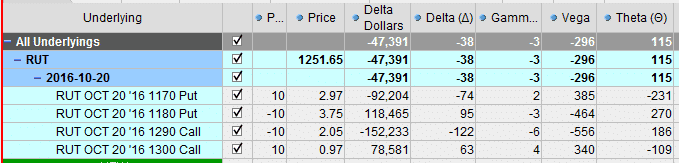You could leave the position as is, but if the market rallies 1-2%, the position will start to be in real trouble.

There are many, many adjustment techniques which I will go over in more detail shortly, but here is one possible scenario.

Close half of the contracts on the under pressure side.

The profit potential on the trade has been lowered, but our Delta to Theta ratio is back to 10% and the trade is much safer now.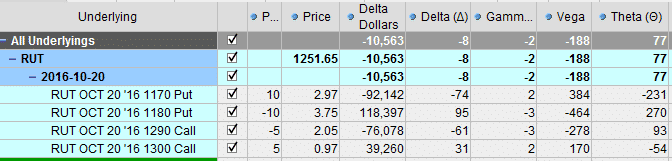## Delta Dollars

Before I move on to the Vega Theta ratio I want to talk a bit about Delta Dollars.

Delta Dollars is calculated as Position Delta x Underlying Price. E.g. -38 x 1251.65 = roughly -47,391.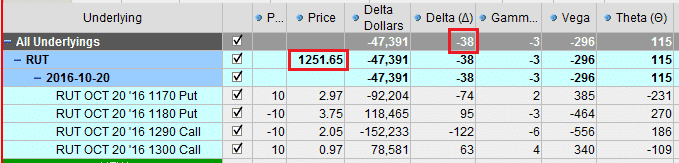Delta Dollars can tell you roughly how much a position can gain or lose depending on how much price moves. It’s not an exact science because of our old friend gamma, but this is the rough calculation.

Price move in percentage x Delta Dollars.

E.g. for this position, if price moves up 1%, the position will lose:

1% x \$47,391 = \$473.91

Similarly, if price moves down 1% the position will gain \$473.91

If price moves up 2% the position will lose roughly:

2% x -\$47,391 = -\$947.82

In actual fact the loss will be larger thanks to gamma as losses will accelerate as the position moves against us.

Note: this calculation assumes no change in volatility, time to expiry or any other variable other than price.

Again, I’ll go over Delta Dollars in much more detail, but for now, start to be aware of this metric.

Rules can be developed to ensure your Delta Dollar exposure doesn’t get too large.

For example, I like to keep my Delta Dollar exposure less than 150% of my capital balance.

E.g. If your capital balance is \$50,000, don’t let Delta Dollars get above plus or minus \$75,000.

## Vega Theta Ratio

The Vega Theta ratio is another important metric to keep an eye on.

When the ratio gets too high, volatility will have a big impact on your position.

We want Theta to be the major driver of our trades and portfolio.

If you have a lot of short Vega trades, like iron condors, your Vega to Theta ratio can be quite high. Adding some long Vega trades can help bring the ratio back in to line.

A good rule of thumb is to keep the Vega to Theta Ratio to less than 400%.

This will vary depending on the strategy, instrument and time to expiry.

In our condor example, the Vega Theta ratio was 257% which is ok.Vega exposure increases as you go further out in time. Theta also decreases.

Therefore, longer term trades will have a much different Vega Theta ratio.

For example, this 60 day iron condor has a Vega Theta ratio of 571%.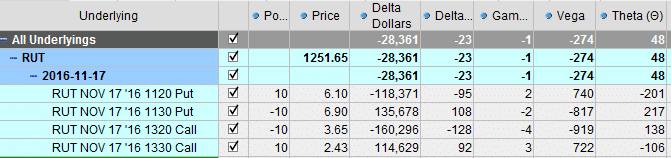A weekly iron condor has a much different ratio again.

Delta Theta ratio is 13%.

Vega Theta ratio is 68%

Both with in acceptable boundaries, but don’t be fooled, this is a very risky trade!

Look at Gamma at -11.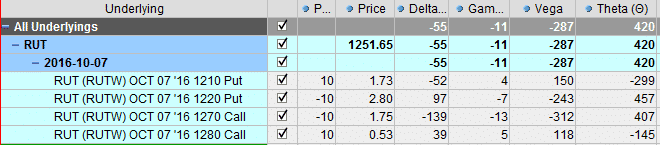## Different Underlyings = Different Rules

The ratios we have looked at so far were all for positions in RUT.

But, the ratios will be different for different underlying instruments.

Look at this trade in GLD for example:

Delta / Theta = 270%

Vega / Theta = 2187%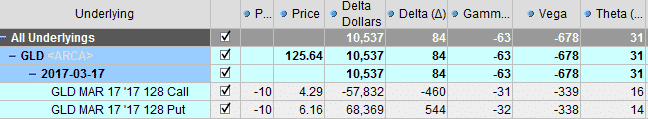Get to know each underlying instrument that you trade and you will soon learn the ratios for each.

Also, keep an eye on Delta Dollars, you can use this to standardize your Delta exposure. More on this shortly.

## Overall Portfolio

As well as looking at the Greeks and Greek Ratios on individual positions, we can also look at them on the overall portfolio.

For example, let’s assume we have a number of positions open in RUT in various strikes.

We determine that our Delta Dollar exposure and Delta / Theta Ratio is too high. We could adjust some of the existing position, OR we could layer in another position that helps bring our exposure down.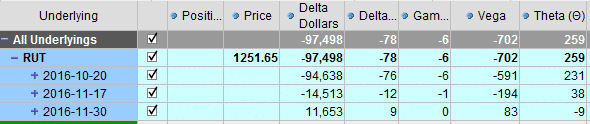Adding an SPX bull put spread, helps bring our Delta Dollar exposure back close to zero and our Greek Ratio are looking much better.